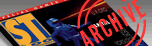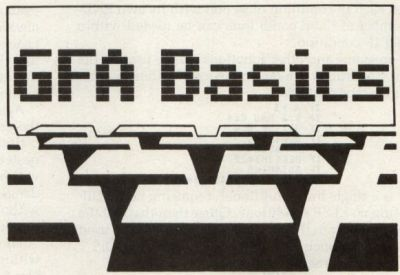`ST-Log ISSUE 18 / APRIL 1988  / PAGE 79`

 PROGRAMMING GFA BasicsBuilding blocks. by Ian Chadwick Ian Chadwick is a Toronto-based free-lance writer and editor who is currently working on a spy novel and a wargame, among other things. The essential element of BASIC programming is the conditional statement—the building block on which most programs depend. GFA provides four conditionals: IF, EXIT IF, REPEAT/UNTIL and WHILE/WEND. Each of these works in a similar fashion, but there are subtle and important differences. Most programmers are accustomed to IF commands. In GFA, the IF command begins a program block that must end with ENDIF. The simplest syntax is: ```IF (condition is true) THEN (do something) ENDIF ``` For example: ```PRINT "Enter a number between 1 and 10 (99 to quit)." Z = RANDOM(9)+1 REPEAT INPUT A IF A = Z THEN PRINT "You guessed the correct number!" END ENDIF UNTIL A = 99 ``` THEN is optional, but worth using for the sake of clarity. If the condition in the IF line isn't true, then the program drops down to the line immediately following the next ENDIF, and everything in between is ignored. Alternatively, you can add additional conditions using the ELSE statement: ```IF (condition is true) THEN (do something) ELSE (do sonething else) ENDIF ``` For example: ```PRINT "Enter a number between 1 and 10 (99 to quit)." Z = RANDOM (9) + 1 REPEAT INPUT A IF A = Z THEN PRINT "Correct! You win!" END ELSE PRINT "You guessed wrong. . . try again." ENDIF UNTIL A = 99 ``` or, you can nest IF statements using ELSE: ```IF (condition is true) THEN (do something) ELSE IF (another condition is true) THEN (do something else) ELSE IF (another condition) THEN (do something) ENDIF ENDIF ENDIF ``` For example: ```PRINT "Enter a number between 1 and 10 (99 to quit)." Z = RANDOM (9) + 1 REPEAT INPUT A IF A = Z THEN PRINT "You guessed the correct number!" END ELSE IF A = Z - 1 PRINT "You are one too low." ELSE IF A = Z + 1 PRINT "You are one too high." ELSE PRINT "Wrong. . . try again." ENDIF ENDIF ENDIF UNTIL A = 99 ``` Note that each IF condition must end with its own ENDIF. Any number of ELSE conditions can be nested within the original IF condition. Logical operators and other functions can be used in the conditions. Conditions can be simple expressions or complex formulae. For example: ```IF A > 3 IF B=3 And C=4 IF A > 1 Or B=6 IF ODD(A) IF A=FALSE IF PEEK(N)=22 IF OUT(0)=0 IF ((A/B)/C)>((D/A)*N) ``` EXIT IF is a single line conditional, requiring no ENDIF and accepting no ELSE conditions. Other than that, all the conditions that apply to IF apply to EXIT IF. EXIT IF is used to get out of loops such as FOR/NEXT and DO/LOOP: ```FOR N = 1 to 1000 PRINT N, EXIT IF N =234 NEXT N ``` or: ```DO INPUT A EXIT IF A = 99 B = B+A LOOP ``` Dropping out of an unfinished FOR/NEXT loop in this fashion seems to have no adverse effect on the program. However, using an IF/ENDIF in a FOR/NEXT loop generates an error message, as in this example: ```FOR N = 1 to 1000 PRINT N, IF N = 234 GOTO CODE2 ENDIF NEXT N ``` Using EXIT IF is the correct way to quit before completion—avoid IF alone to escape loops. REPEAT/UNTIL and WHILE/WEND both allow a single conditional to determine iterations of a program block: ```REPEAT (program code) UNTIL (condition) ``` For example: ```PRINT "Enter a number between 1 and 10 (inclusive)." Z = RANDOM(9)+1 B = 8 REPEAT PRINT "Try again!" INPUT A ADD B, 1 PRINT "Guesses =";B UNTIL A = Z PRINT "Correct in ;"B;" guesses!" ``` and: ```WHILE (condition) (program code) WEND ``` For example: ```PRINT "Enter a number between 1 and 10 (inclusive)." Z = RANDOM (9)+1 B = 0 WHILE A <> Z PRINT "Try again!" INPUT A ADD B, 1 PRINT "Guesses =";B WEND PRINT "Correct in ;"B;" guesses!" ``` The difference between the two is where the condition is checked. In REPEAT/UNTIL blocks, it is checked at the end of the iteration, allowing other functions and commands to take place until the UNTIL line is reached (in the last example, B is incremented). The program block is therefore always executed at least once before the condition is checked. In WHILE/WEND blocks, since it is checked before the code is executed, the code will not be executed if the condition is true (B is not incremented in the last example). Again, conditions that apply to the IF function apply to the conditions stated in UNTIL and WHILE. Both REPEAT/UNTIL and WHILE/WEND loops can be nested within themselves and each other, not to mention within IF/ENDIF, DO/LOOP and FOR/NEXT loops. The danger, of course, is in the ability to create endless loops without exits—so use the EXIT IF command, at least, to provide a proper escape. The other caution is to be careful when mixing variables within loops. Unlike procedures, there are no local variables for loops, only global variables, so it's easy to get confused. Small plug. MichTron runs a conference section on GEnie, the online database (this is separate from their own in-house BBS). Although not strictly limited to GFA BASIC, their database of download-able files includes many utilities, programming examples, games and demos. They also have a weekly online conference every Sunday about GFA, in which the MichTron technical staff participate along with any users—you get news, gossip, comments, questions, and so on. It's pretty free-form. One of the more difficult (in terms of complexity) tasks in GFA is creating dialog boxes—there is no equivalent command as there is for alerts. However, there are dialog box creation programs for medium/high and low resolutions in their GEnie database (the archived files also include examples). I recommend you get them if you want to make the process a lot easier. A lot of good information is to be had in both the conference and the database. The conferences are archived into files in the GEnie database after a week or so, if you missed one. I haven't seen these programs on any other service, although I have seen other programs on CompuServe and Delphi.